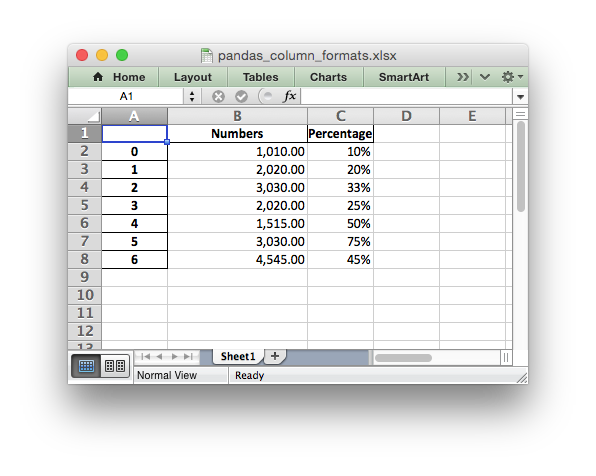# Example: Pandas Excel output with column formatting#

An example of converting a Pandas dataframe to an Excel file with column formats using Pandas and XlsxWriter.

It isn’t possible to format any cells that already have a format such as the index or headers or any cells that contain dates or datetimes.

Note: This feature requires Pandas >= 0.16.```##############################################################################
#
# An example of converting a Pandas dataframe to an xlsx file
# with column formats using Pandas and XlsxWriter.
#
# Copyright 2013-2023, John McNamara, jmcnamara@cpan.org
#

import pandas as pd

# Create a Pandas dataframe from some data.
df = pd.DataFrame(
{
"Numbers": [1010, 2020, 3030, 2020, 1515, 3030, 4545],
"Percentage": [0.1, 0.2, 0.33, 0.25, 0.5, 0.75, 0.45],
}
)

# Create a Pandas Excel writer using XlsxWriter as the engine.
writer = pd.ExcelWriter("pandas_column_formats.xlsx", engine="xlsxwriter")

# Convert the dataframe to an XlsxWriter Excel object.
df.to_excel(writer, sheet_name="Sheet1")

# Get the xlsxwriter workbook and worksheet objects.
workbook = writer.book
worksheet = writer.sheets["Sheet1"]